# OFDM Channel Estimation (1)¶

In the previous notebook, we have managed to perform coarse timing synchronization to extract the actual OFDM symbols from the received signal. In addition, we have seen that the coarse timing is not enough to let us show a decent constellation diagram, as an erroneous timing by already one sample will destroy the constellation.

In this notebook, we will introduce the most basic OFDM channel estimation technique to finally be able to show a correct constellation diagram.

Before we go into the implementation of the channel estimation, let us shortly reconsider the fundamentals of channel estimation. In this course, we focus on data-aided channel estimation, which means we include known signals (named pilots) into the transmit signal which we evaluate at the receiver side. By looking at how these known signals have changed when they were sent over the channel, we can estimate the effect of the channel. We can then use this estimate to equalize the remaining, unknown OFDM symbols.

Let's quickly go through the mathematical fundaments for channel estimation: In OFDM, in the frequency domain, the received signal $Y[k]$ is given by

$$Y[k]=H[k]X[k] + N[k]$$

where $X[k]$ is the transmitted signal on the $k$th carrier and $H[k]$ is the channel response on the $k$th carrier. $N[k]$ is the noise on the $k$th carrier. At the receiver, we know $Y[k]$. To also estimate $X[k]$ we need to know $H[k]$. To get to know $H[k]$ is the task of the channel estimation.

Now, assume that for some $k_p$, we know the transmitted value $X[k_p]$. In this case, we can estimate $H[k_p]$ by

$$H[k_p] = Y[k_p] / X[k_p]$$

We call the $k_p$ where we know $X[k_p]$ the pilot carrier and $X[k_p]$ is called the pilot. Naturally, the more pilots we place into the signal, the more can we estimate about $H$ and the more accurate can we know $H$. On the other side, pilot carriers cannot be used for data transmission and hence we reduce the available data rate when we put more pilots into the signal. This tradeoff between pilot overhead and channel estimation quality is common in any wireless communication system.

Depending on the application, we have different possibilities to place the pilots within the OFDM symbol:

In :
# Source code has been redacted in online version
# Omitting 36 lines of source codeWith Block-type pilots, one OFDM symbol is full of pilots, i.e. each carrier contains a known value. These pilots are then used to estimate the channel on each carrier, and subsequent OFDM symbols are equalized using these estimated channel coefficients. Block-type pilots are similar to preamble-based channel estimation in the sense that by knowing the value on each carrier gives us full knowledge of the OFDM symbol in time domain, hence it can be considered as a known preamble. The estimation using block-type is most simple, as we do not need to interpolate the estimate to different carriers. In addition, block-type pilots provide a high accuracy in the frequency domain, as we have many measurements in one estimation. Though, block-type pilots are not useful for quickly time-varying channels due to less resolution in the time domain.

With Comb-type pilots several carriers are exclusively used for pilot transmission in each OFDM symbol. Here, frequency-domain interpolation is required to estimate the channel coefficients on non-pilot subcarriers. Comb-type pilots provide a high resolution in the time domain, but less accuracy over the frequency domain. Hence, they are applicable for time-varying channels.

Using Scattered pilots one seeks a tradeoff between time-resolution, frequency resolution and pilot overhead. Scattered pilot schemes require interpolation schemes in the time- and frequency domain, but can deliver a better tradeoff in accuracy in time and frequency. Scattered pilots are used for example in the celluar LTE system.

## OFDM Frame Structure¶

In this notebook, we will aim for the most simple channel estimation method and hence choose a block-type pilot placement. Specifically, we will create a frame structure that looks as follows:

In :
# Source code has been redacted in online version
# Omitting 11 lines of source codeThe first OFDM symbol in the frame starts with the preamble that is used for Schmidl&Cox synchronization. The second symbol contains the block-type pilot signal. All remaining OFDM symbols contain the unknown payload that should be decoded at the receiver side. In the most basic scheme, we use the pilots from the second symbol to estimate the channel that is used to equalize any subsequent payload symbol.

Let's get started with the implementation. First, we import some commont objects:

In :
from audioComms.components import Component, TX, Environment, Recorder
from audioComms.plotting import PlotWaveform, PlotSpectrum, PlotConstellation, PlotBlock
from audioComms.channels import AudioChannel, SimulatedChannel
from audioComms.channels import IdentityChannelEffect, SimulatedChannelEffect, NoiseChannelEffect, InitialLossChannelEffect, ChainedChannelEffects, LoseSamplesChannelEffect

from audioComms.passband import PassbandChannel


Moreover, we import OFDM-specific functions we have defined in the previous notebooks:

In :
from audioComms.ofdm import OFDM, random_qam, ofdm_modulate, MostBasicOFDMReceiver, createSchmidlCoxPreamble
from audioComms.synchronization import SchmidlCoxDetectPeaks, SchmidlCoxMetric


Let us now start with the OFDM signal generator. An important part is the block-type pilot symbol. As described before, the transmitted pilot values need to be known at the receiver. Hence, we cannot transmit random values. Instead, we choose a fixed pilot value sequence. A common choice for pilot values are Zadoff-Chu-sequences due to their nice correlation properties, which can in addition be used for synchronization purposes.

Here's a function to create the ZC-sequence:

In :
def ZadoffChu(order, length, index=0):
cf = length % 2
n = np.arange(length)
arg = np.pi * order * n * (n+cf+2*index)/length
return np.exp(-1j*arg)


Moreover, given that we do not use all carriers of an OFDM symbol (i.e. $K_{on} In : def get_carrierIndices(ofdm): off = int(np.ceil((ofdm.K - ofdm.Kon)/2)) return np.arange(ofdm.Kon) + off  Let's quickly look at an example result, with an OFDM symbols with 16 carriers, where only 8 of them are allocated. In : ofdm = OFDM(K=16, Kon=8) print (get_carrierIndices(ofdm)) carriers = np.arange(ofdm.K) carriers_on = np.zeros(ofdm.K); carriers_on[get_carrierIndices(ofdm)] = 1 plt.figure(figsize=(8,2)); plt.stem(carriers, carriers_on); plt.ylabel('Subcarrier allocated?'); plt.xlabel('Subcarrier index'); plt.grid(True); plt.ylim((-0.1,1.1));  [ 4 5 6 7 8 9 10 11]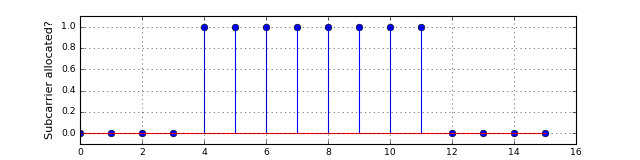Ok, clearly the center carriers, i.e. the carriers around the DC frequency are allocated. We are now ready to create our OFDM transmitter. The signal generation simply follows the frame structure we have defined before. To be able to distinguish between different frames, we artificially insert zeros between them: In : # Source code has been redacted in online version # Omitting 15 lines of source code  Now, let's look at the TX signal: In : def showTxSignal(): env = Environment(samplerate=1000, simulationDuration=5) ofdm = OFDM(K=256, Kon=200, CP=64, Npayload=3) tx = OFDMTransmitter(env, ofdm) channel = SimulatedChannel(env) showSig = PlotWaveform(env, figsize=(10,3), signalTransform=abs, windowDuration=2, ylim=(-0.1, 2)) tx.transmitsTo(channel) channel.transmitsTo(showSig) env.run() showTxSignal()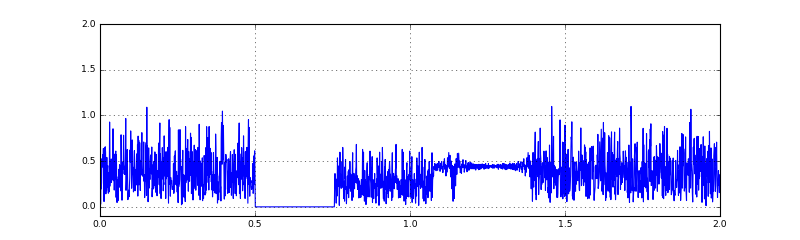Ok, we cearly see the different parts of the frame: First, we have the S&C-preamble, then we have a special structure containing the pilots and finally we get the actual payload symbols. Let us now switch over to the receiver side. In the previous notebooks we have already created the synchronization block, so we focus on the OFDM demodulation block. The receiver receives an extra object that will perform the actual channel estimation. Channel equalization is then performed in the receiver. The receiver object creates several debug streams which can be used to display some informative plots. In : # Source code has been redacted in online version # Omitting 37 lines of source code  The above OFDMReceiver block requires a ChannelEstimator block. This is what we are going to define next. Let's define the basic interface: In : class ChannelEstimator(object): def __init__(self, ofdm): self._ofdm = ofdm # pre-compute the pilot values and corresponding allocated carriers self._pilotVals = ZadoffChu(length=ofdm.Kon, order=1) self._carrierIndices = get_carrierIndices(self._ofdm) def estimate(self, pilotBlock): # this function should perform the estimation raise NotImplementedError()  Now that we have defined the interface, let's implement the most basic one: A simple Leeast-squares estimator that is based on the simple division by the channel. Since we have a pilot on each carrier, we do not even need to perform interpolation or similar. In : class MostBasicChannelEstimator(ChannelEstimator): def estimate(self, pilotBlock): # obtain the received pilot values pilots = np.fft.fftshift(np.fft.fft(pilotBlock)) usefulPilots = pilots[self._carrierIndices] # LS estimate of the channel Hest = usefulPilots / self._pilotVals return Hest  We are now ready to chain everything together to finally see our constellation diagram: In : # Source code has been redacted in online version # Omitting 42 lines of source code  Let's first run the system in the noiseless, ideal, simulated channel: In : runTransmission(SimulatedChannel)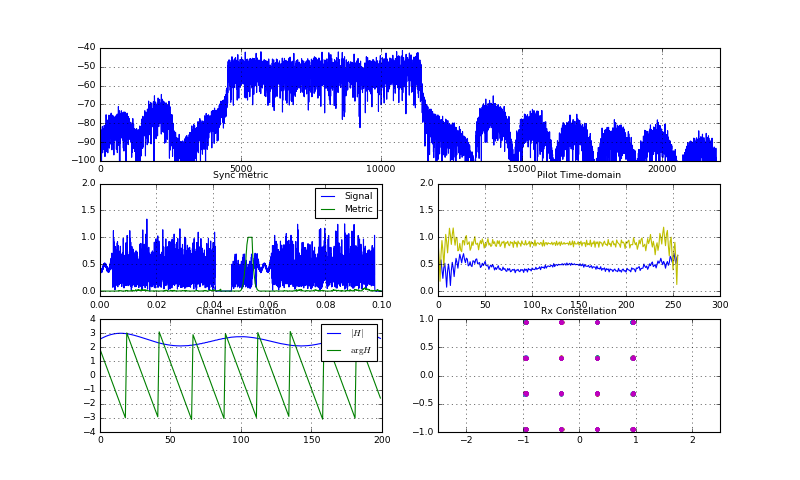Stop received from external  Cool! We can clearly see a decent constellation diagram with very sharp constellation points. In addition, the sync metric is very clear, but what's going on in the output of the channel estimation? First, let's look at the magnitude (blue curve). We see a slight variation of the magnitude over the frequency domain. This variation most probably comes from our method of up- and downconversion rather than an actually frequency-selective channel. However, in general the channel appears almost flat. But, what's up with the phase? The phase is decreasing, so the channel is roughly equal to $$H[k] = \exp(-j2\pi k \Delta_\phi/K)$$ if we assume the magnitude is constant. Here,$\Delta_\phi$is the number of phase wraparounds. What does that correspond to in the time domain? $$h[n] = \text{IDFT}_K\{H[k]\} = \delta[n-\Delta_\phi]$$ It's a unit impulse shifted to the right by the number of wrap-arounds. This exactly shows us the error of the coarse time-synchronization. Luckily, our channel equalization does compensate for it. Now, let's move on to see if it actually works in the audio channel (if you cant see a constellation, play around with the volume and try a cable first): In : runTransmission(channelFunc=AudioChannel)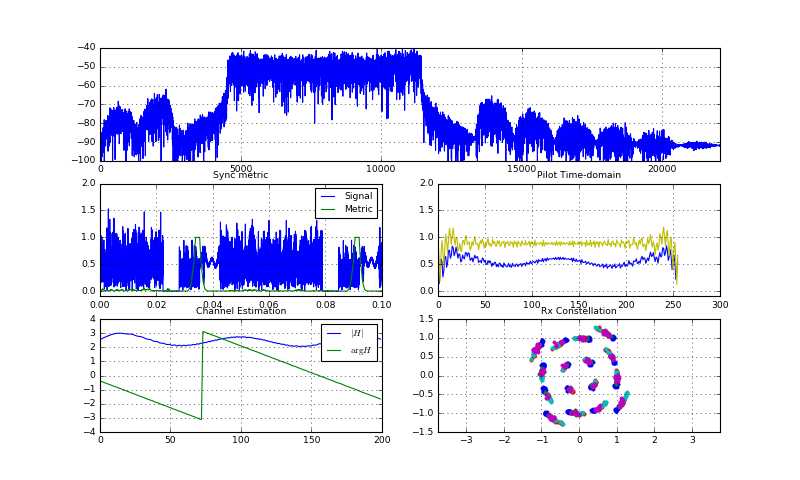Stop received from external Stream Stopped 1 Stream Stopped 2  Yes, it also works and we see a constellation diagram. However, the points are not as sharp as with the simulated channel. First, the constellation position constantly changes from frame to frame. Also, all points seem to be rotated a bit and finally, within each constellation point, there seems to be a circular movement of the points. However, the constellation points from one OFDM symbol (i.e. one color) seem to be all clustered together, but between the different symbols there is a rotation. Let us investigate what is going on there. Note: Depending on your soundcard, you may not see the effect I describe here. Read on to see a simulation of the effect. First, let us run the same system, but with less bandwidth, i.e. the symbols become longer with narrower subcarriers: In : runTransmission(channelFunc=AudioChannel, B=441*5)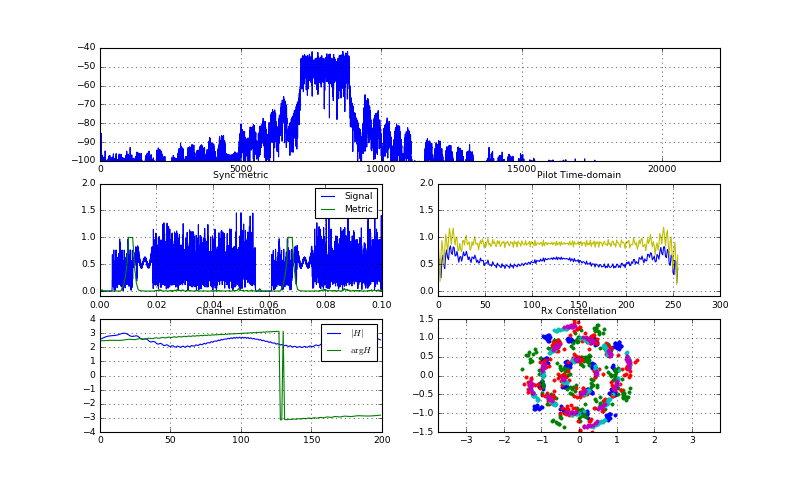Stop received from external Stream Stopped 1 Stream Stopped 2  Uh, the effect of the rotation of the symbols becomes even stronger! But, again, within one OFDM symbol the points seem to be clustered together. However, they are not as sharp as before anymore. Clearly, we see some time-varying property of the audio channel. We can conclude this, since different OFDM symbols create a different rotation in the constellation plane and hence experience a different channel. If we talk about time-varying channels, there are (at least) two possibilities: 1) The channel changes since the antenne geometry changes, i.e. the microphone is moved or objects in the vicinity move, creating different reflections. 2) There is a frequency mismatch between TX and RX which creates time-variation. Since only the phase of the channel seems to change, let's quickly simulate what happens when we apply a slight frequency offset to the system: In : runTransmission(channelFunc=SimulatedChannel, B=441*5, cfo=.2) # add 0.2Hz frequency offset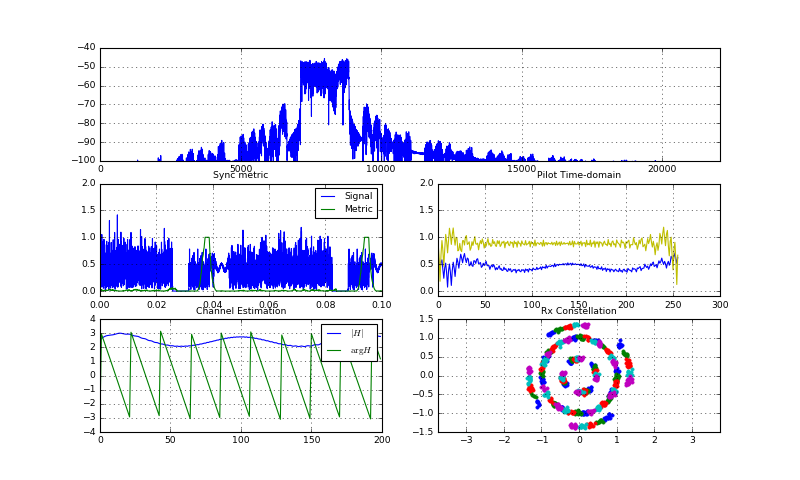Stop received from external  Yes! With the CFO addition, we get a similar channel effect as in the audio channel. Let us for now assume that our soundcard also produces a similar frequency offset. In the following notebook, we will proceed to estimate and compensate the frequency offset to analyze in more detail what the soundcard is doing. For now, let's do a short calculation: In the above system, our system bandwidth is$B=441*5Hz=2205Hz$, which is divided into$256$subcarriers. Hence, the subcarrier spacing equals$B/256=8.6Hz$and a$0.2Hz$frequency offset hence equals 2.3% of a subcarrier spacing. Now, let's again run the same system with a larger bandwidth and hence carrier spacing: In : runTransmission(channelFunc=SimulatedChannel, B=441*5*4, cfo=.2)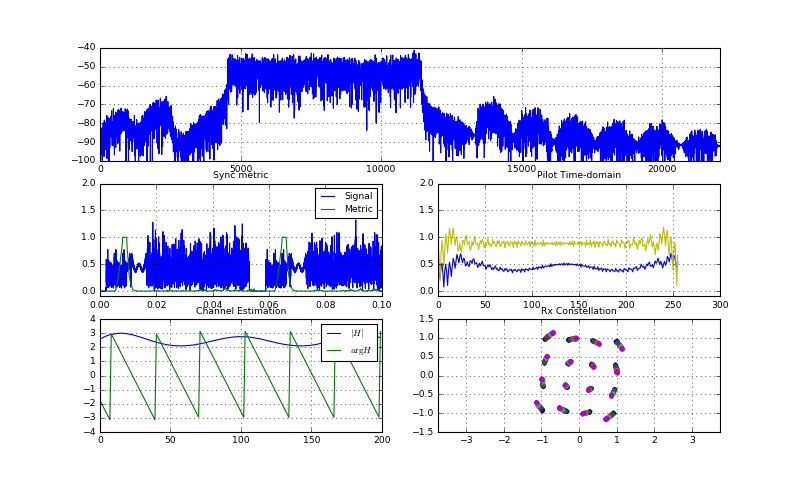Stop received from external  Clearly, the effect of the cfo is reduced, in two ways: • the constellation points become sharper lines • there is less rotation between the points. Clearly, we can explain this as follows: We have a bandwidth of$B=441*5*4Hz=8820Hz$and hence the subcarrier spacing equals$B/256=34.4Hz$and therefore$0.2Hz\$ frequency offset is only 0.58% of the carrier spacing. Hence, the effect of the CFO is much less pronounced.

## Summary¶

In this notebook, we have finally been able to obtain an acceptable constellation diagram using the audio channel. We have combined the coarse time synchronization from Schmidl&Cox and the fine-timing coming from the block-type channel estimation to find the correct timing and apply a simple channel equalization to obtain the correct constellations.

However, we have experienced that our soundcard seems to produce some frequency offset and hence the constellation points were not very clear. In the following notebook, we will extend the Schmidl&Cox synchronization scheme to additionally estimate frequency offset and subsequently cancel it.